Product category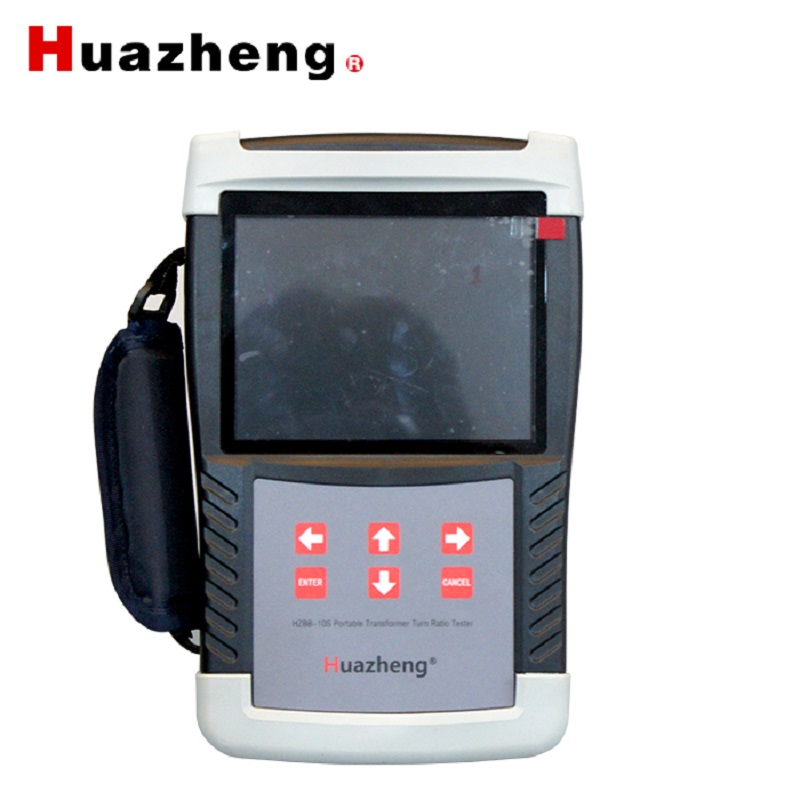HZBB-10S turns ratio tester transformer ratio testing equipment automatic transformer turns ratio meter ttr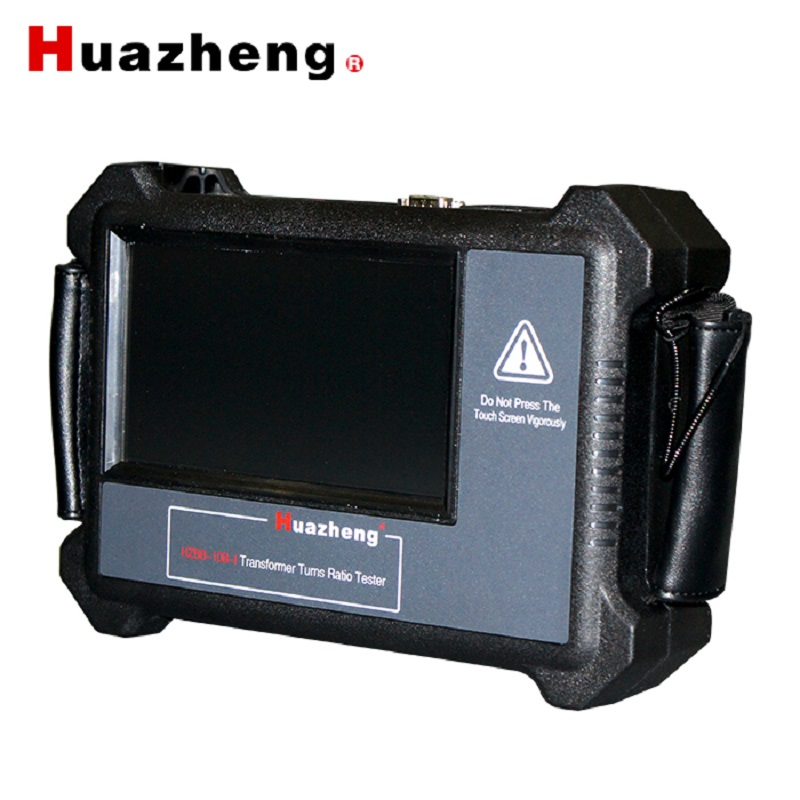HZBB-10B-I Turns Ratio Tester Turns Ratio Tester Portable TTR Meter Transformer Turns Ratio Analysis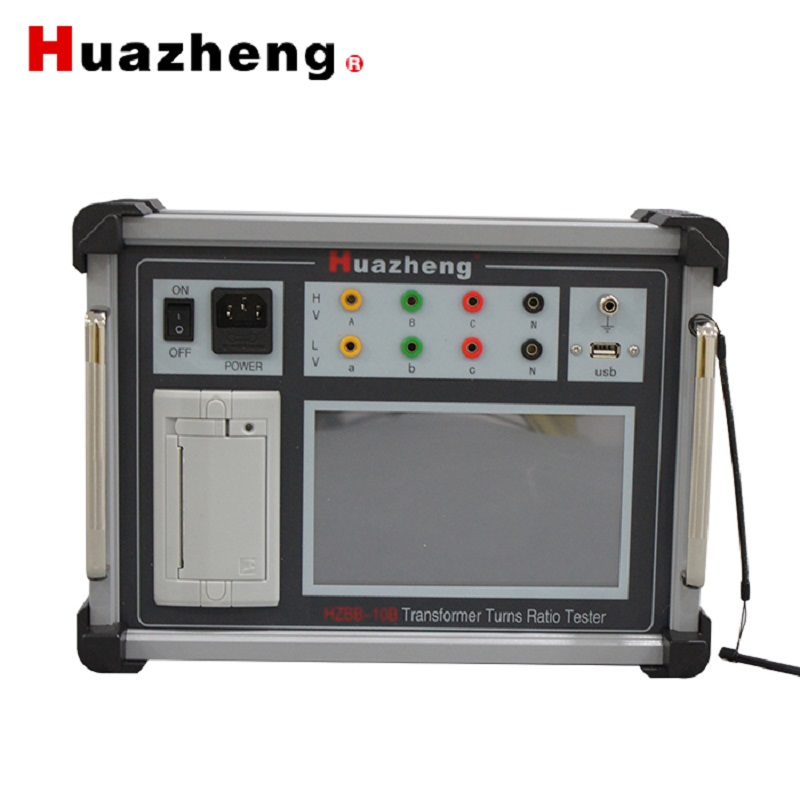HZBB-10B ttr testing equipment turns ratio tester transformer turns ratio meter ttr digital transformer turns ratio meter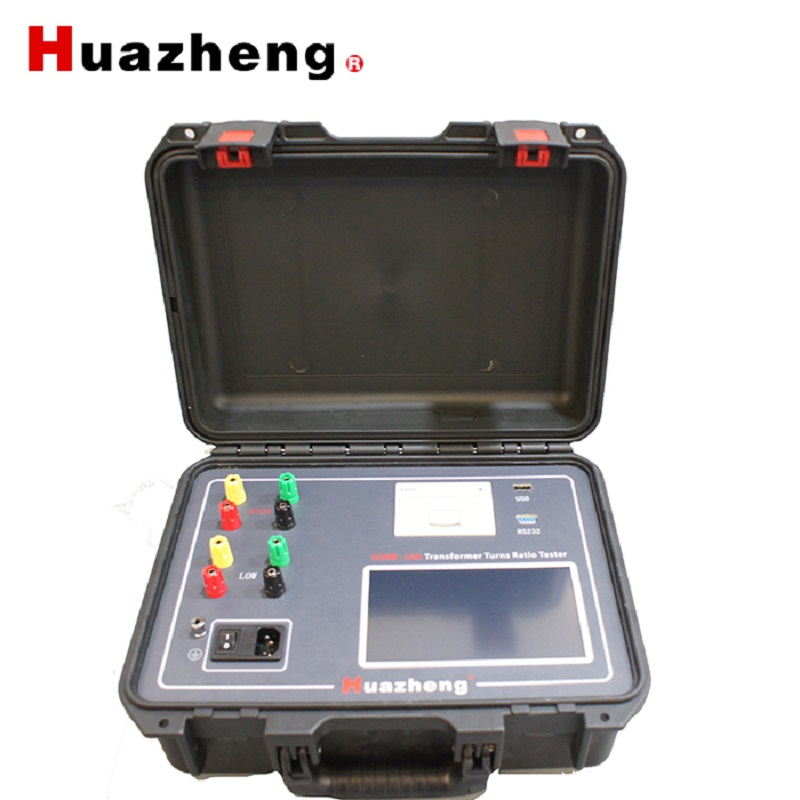Huazheng Electric HZBB-10A Turns Ratio Group Tester Turns Ratio Tester Transformer TTR Tester Equipment Electric Test Turn Ratio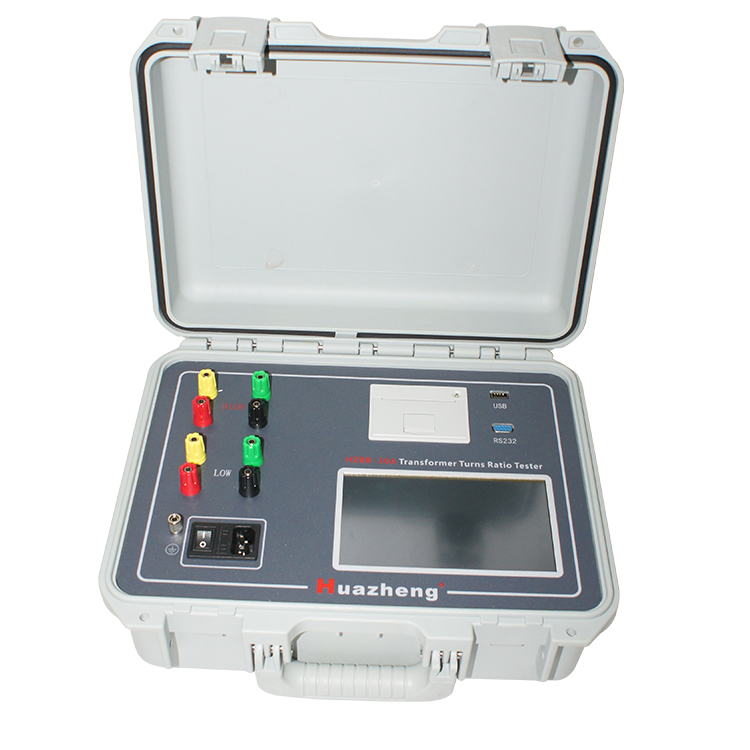Huazheng Electric Fully Auto 0.9-10000 3 phase transformer turns ratio group tester digital display turns ratio tester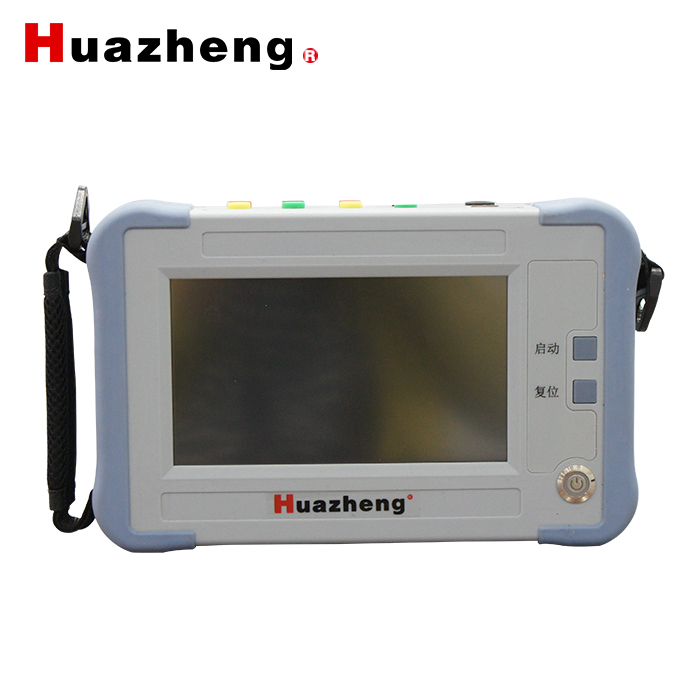HZBB-10A-I Hand -held transformer turns ratio tester digital transformer turns ratio meter portable ttr meter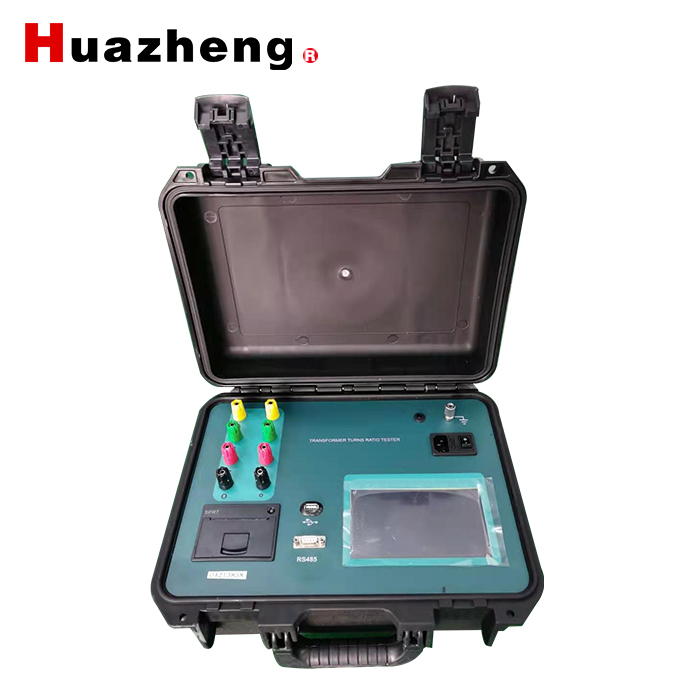HZBB-10H Transformer Turns Ratio Tester TTR Turns Ratio Test Device Transformer Turns Ratio Analysis Huazheng Electric TTR testing equipment